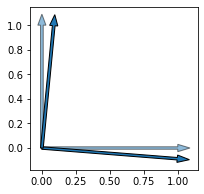# Rotation matrix

Hi, lets think matrix x = Torch.rand((256,2)). How can I rotate this matrix in PyTorch ? For example by 5 degrees. How to create rotation matrix for this ?

Thank you

1 Like

So you have 256 points in 2d?

``````import math
x = torch.rand(256, 2)
phi = torch.tensor(5 * math.pi / 180)
s = torch.sin(phi)
c = torch.cos(phi)
rot = torch.stack([torch.stack([c, -s]),
torch.stack([s, c])])

x_rot = x @ rot.t()   # same as x_rot = (rot @ x.t()).t() due to rot in O(n) (SO(n) even)
``````

or somesuch.

Best regards

Thomas

2 Likes

Thank you a lot, this helped me!

1 Like

If I can have one more question what is the difference betwen-

``````x_rot = x @ rot.t()
``````

and

``````x_rot = torch.matmul(x,rot)
``````

`@` and `torch.matmul` are identical, but if you leave out the `.t()`, you’ll change the direction of rotation (because `.t()` is the inverse rotation), I thought that the version with `.t()` might be the more canonical (because the convention would seem to be to multiply from the left but that doesn’t work with the batch dimension coming first). Combined this is the funny math geek comment in the code snippet.2 Likes

@tom Hi, one more thing. Do you have any idea how to plot these two matrices and see rotation on some type of plot ? When I want to do this i cannot just plot Matrix as image right ?

Maybe plotting one or two rotated unit vectors (ie use the two entries of a row of the matrix as x and y) would work.

Edit: Back at the computer (and “eer” about the rotation direction convention…):

``````from matplotlib import pyplot
pyplot.figure(figsize=(3, 3))
pyplot.arrow(0, 0, 1, 0, width=0.02, alpha=0.5)
pyplot.arrow(0, 0, 0, 1, width=0.02, alpha=0.5)
pyplot.arrow(0, 0, rot[0, 0].item(), rot[0, 1].item(), width=0.02)
pyplot.arrow(0, 0, rot[1, 0].item(), rot[1, 1].item(), width=0.02)
``````1 Like